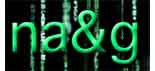Today I
did prepare my lectures for tomorrow for the NOG master-class on
non-commutative geometry. I\’m still doubting whether it is worth TeXing
my handwritten notes. Anyway, here is what I will cover tomorrow :

– Examples of l-algebras (btw. l is an
arbitrary field) : matrix-algebras, group-algebras lG of finite
groups, polynomial algebras, free and tensor-algebras, path algebras
lQ of a finite quiver, coordinaterings O(C) of affine smooth
curves C etc.
– Refresher on homological algebra : free and
projective modules, exact sequences and complexes, Hom and Ext groups
and how to calculate them from projective resolutions, interpretation of
Ext^1 via (non-split) short exact sequences and stuff like that.
– Hochschild cohomology and noncommutative differential forms.
Bimodules and their Hochschild cohomology, standard complex and
connection with differential forms, universal bimodule of derivations
etc.
– Non-commutative manifolds. Interpretation of low degree
Hochschild cohomology, characterization of non-commutative points as
separable l-algebras and examples. Formally smooth algebras
(non-commutative curves) characterised by the lifting property for
square-free extensions and a proof that formally smooth algebras are
hereditary.

Next week I will cover the representation
varieties of formally smooth algebras and the semigroup on their
connected (or irreducible) components.

Published in featured